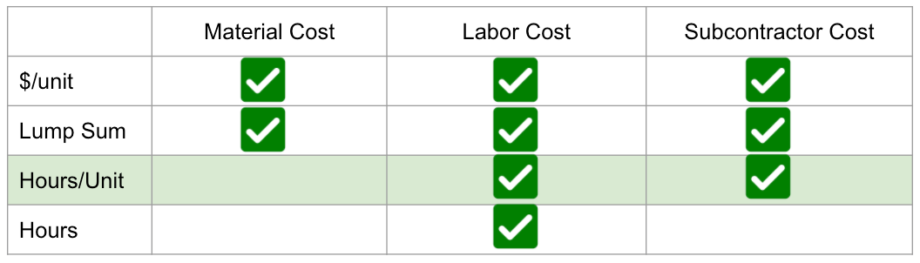# How the Hours/Unit Cost Calculation Works

### Overview

Hours/Unit is great for when you want to charge a customer for a certain number of hours on a per unit basis. This is perfect for installation costs - when you know how long each unit will take, and the labor rate you want to charge. This is the default calculation in Clear Estimates for Labor Costs.

Enter the number of hours per unit (i.e. 2 hours per square foot), as well as the labor rate to charge (i.e. \$30/hr). Then, Clear Estimates will use that rate and the quantity (# of square feet) to calculate the total labor cost for the part.

(Hours/Unit * Labor Rate) * Quantity = Labor Cost

### Where You Can Use It

The hours/unit calculation option is available for your Labor or Subcontractor costs! That means you can set 3 Hours per Piece at \$45/hr for Labor or 0.5 hours per square foot at \$100/hr for Subcontractor Costs! Or, any combination of the two!

#### What costs can be hours/unit?### Examples

Charge a Hours/Unit for Labor

Charging your customer a hours/Unit for labor is easy and is the default way to calculate labor costs in Clear Estimates!

Just open the part and make sure the "Labor Cost Calculation" is included for this part.

Then select the "Hours/Unit" calculation type for the labor costs.

Last, simply enter the hours for each unit (how long it will take per unit) and the labor rate!

You can use the same process for Subcontractor Costs as well!